# 1) (18 points) A first-order reaction A B begins at 8:30AM with [A] -0.45 M. If[A]...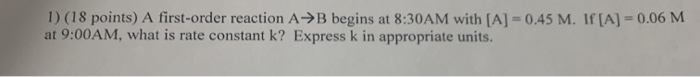1) (18 points) A first-order reaction A B begins at 8:30AM with [A] -0.45 M. If[A] = 0.06 M at 9:00AM, what is rate constant k? Express k in appropriate units.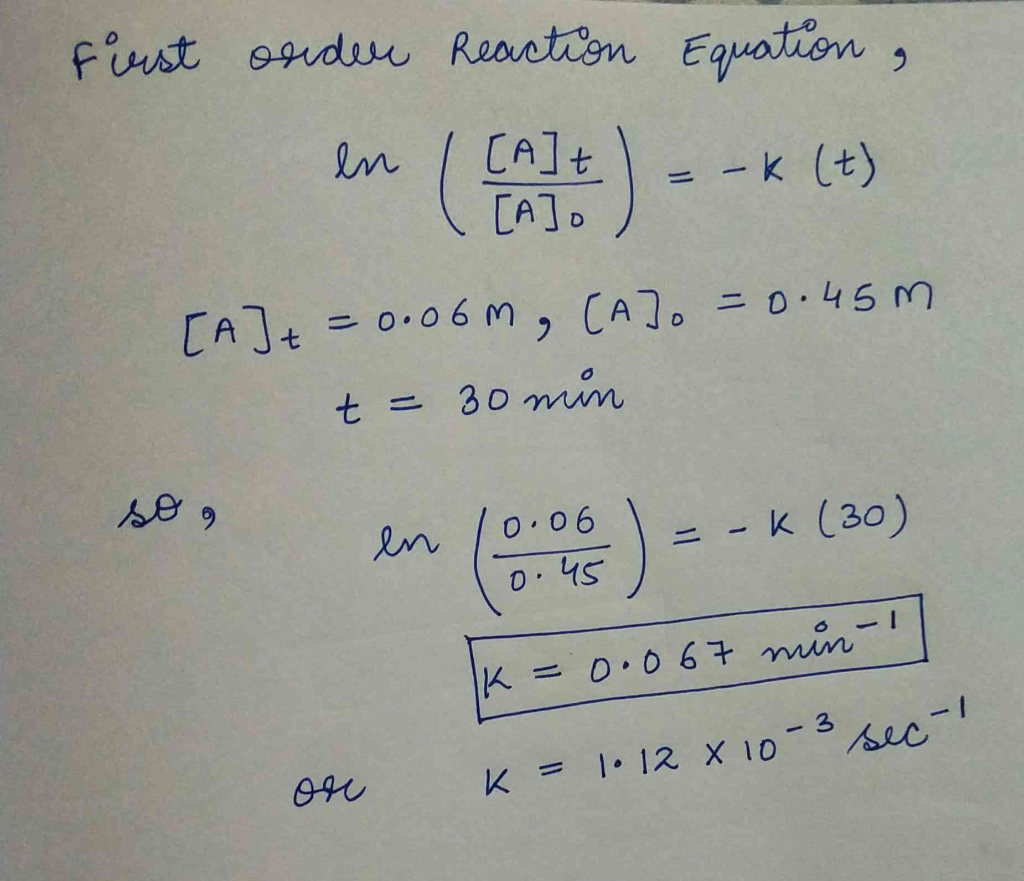##### Add Answer of: 1) (18 points) A first-order reaction A B begins at 8:30AM with [A] -0.45 M. If[A]...
Similar Homework Help Questions
• ### 2) (18 points) A second-order reaction A B begins at 11:30AM with [A] 0.75 M. If...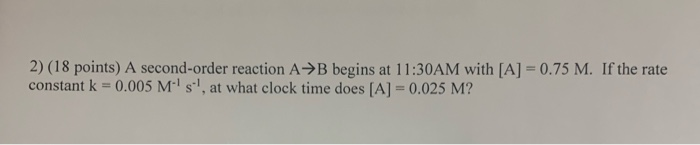2) (18 points) A second-order reaction A B begins at 11:30AM with [A] 0.75 M. If the rate constant k 0.005 M s, at what clock time does [A] = 0.025 M?

• ### + Using Integrated Rate Laws The integrated rate laws for zero-, first-, and second-order reaction may...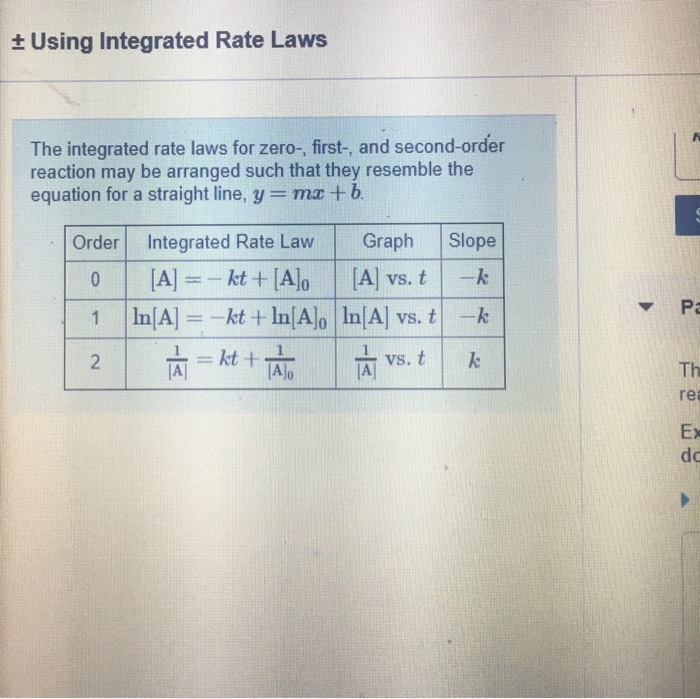+ Using Integrated Rate Laws The integrated rate laws for zero-, first-, and second-order reaction may be arranged such that they resemble the equation for a straight line, y = mx + b. Slope Order O 1 2 Integrated Rate Law Graph [A] = - kt + [A] [A] vs. t In[A] = -kt + In[A], In[A] vs. t LÀ=kt + TA LÀ vs. t -k Review Constants Periodic Table Part A The reactant concentration in a zero-order reaction was...

• ### + Using Integrated Rate Laws The integrated rate laws for zero-, first-, and second-order reaction may be arrang...+ Using Integrated Rate Laws The integrated rate laws for zero-, first-, and second-order reaction may be arranged such that they resemble the equation for a straight line, y = mx + b. Slope Order O 1 2 Integrated Rate Law Graph [A] = - kt + [A] [A] vs. t In[A] = -kt + In[A], In[A] vs. t LÀ=kt + TA LÀ vs. t -k Review Constants Periodic Table Part A The reactant concentration in a zero-order reaction was...

• ### ran The reactant concentration in a first-order reaction was 5.90x10-2 M after 15.0 s and 3.70x10-3...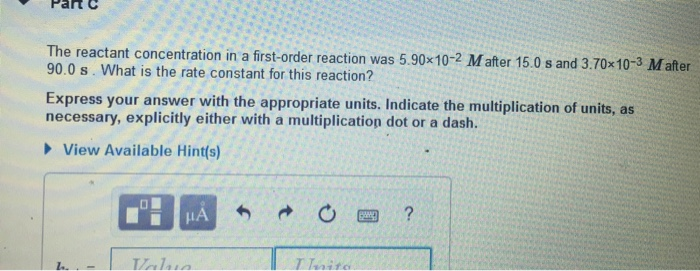ran The reactant concentration in a first-order reaction was 5.90x10-2 M after 15.0 s and 3.70x10-3 M after 90.0 s. What is the rate constant for this reaction? Express your answer with the appropriate units. Indicate the multiplication of units, as necessary, explicitly either with a multiplication dot or a dash. ► View Available Hint(s) THHA O a ? Turita

• ### 18. From the reaction data below, determine whether the reaction is first-order or second-order. Time (S)...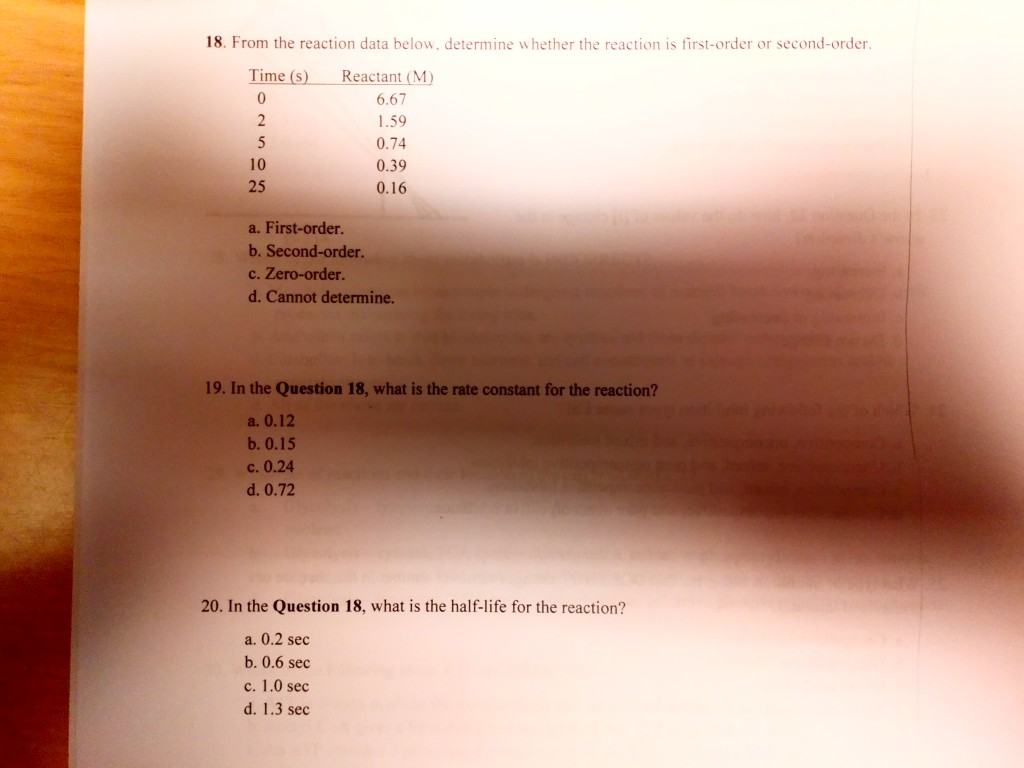18. From the reaction data below, determine whether the reaction is first-order or second-order. Time (S) Reactant (M) 6.67 1.59 0.74 0.39 0.16 25 a. First-order. b. Second-order. c. Zero-order. d. Cannot determine. 19. In the Question 18, what is the rate constant for the reaction? a. 0.12 b. 0.15 c. 0.24 d. 0.72 20. In the Question 18, what is the half-life for the reaction? a. 0.2 sec b. 0.6 sec c. 1.0 sec d. 1.3 sec

• ### 8. Consider the reaction: A → B The rate of the reaction is 1.6 × 10−2 M/s when the concentration of A is 0.35 M. Calcul...

8. Consider the reaction: A → B The rate of the reaction is 1.6 × 10−2 M/s when the concentration of A is 0.35 M. Calculate the rate constant if the reaction is first order in A. Enter only the numerical value for the rate constant in the answer box. 9. The reaction 2A → B is second order with a rate constant of 51.0/M·min at 24°C. (a) Starting with [A]0 = 9.50 × 10−3M, how long will it take...

• ### First Order Reaction/K value

A)A certain first-order reaction has a rate constant of 2.10×10-2 s^-1 at 22 degrees C. What is the value of K at 58 degrees c if Ea = 87.0 Kj/Mol ?B)A certain first-order reaction has a rate constant of 2.10×10-2 S^-1 at 22 Degrees C. What is the value of K at 58 degrees C if Ea = 104 Kj/Mol ?

• ### first-order reaction 1

the rate constant of a first-order reaction is 4.60x10^-4 at 728 K. if the activation energy is 104kJ/mol calculate the temp in K at which its rate constant is8.80x10^-4.

• ### Part A) A certain first-order reaction has a rate constant of 2.40×10−2 s−1 at 25 ∘C....

Part A) A certain first-order reaction has a rate constant of 2.40×10−2 s−1 at 25 ∘C. What is the value of k at 60 ∘C if Ea = 74.0 kJ/mol ? Express your answer using two significant figures. Part B) Another first-order reaction also has a rate constant of 2.40×10−2 s−1 at 25 ∘C. What is the value of k at 60 ∘C if Ea = 135 kJ/mol ? Express your answer using two significant figures.

• ### The rate constant for the first-order reaction below is 0.0840 s–1. \$\$ A B If the...

The rate constant for the first-order reaction below is 0.0840 s–1. \$\$ A B If the reaction is begun with an initial concentration of A equal to 0.95 M, what is the concentration of A after 17.01 s?

Need Online Homework Help?